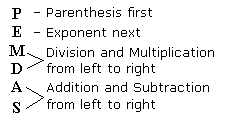# PHP Tutorial 4 – Basic Math OperatorsIn our last tutorial, we discussed variables and comments in PHP. Today, we are going to go over basic math operators. By math operators, I mean symbols that represent math operations. Adding, subtracting, dividing, and multiplying are all examples of math operators. There are seven main operators in PHP, and they can be seen in the following table:

 Operation Operator (Symbol Used To Perform The Operation) Addition + Subtraction – Multiplication * Division / Remainder / Modulus % Increase By 1 / Increment ++ Decrease By 1 / Decrement —

Let’s put the first five basic math operations to use in a short program:

 ``` < ?php echo (6 + 4).""; echo (6 - 4).""; echo (6 * 4).""; echo (6 / 4).""; echo (6 % 4).""; ?> When compiled, the above code will output the following to the screen: 10 2 24 1.5 2 echo (6 + 4).””; Every line of PHP code in this program was almost the same, so let’s take a look at this one. We outputted the sum of six and four to the screen. Notice the lack of quotation marks, this isn’t a string. We also concatenated an HTML line break tag onto the end of our echo function. Without a line break, all of the numbers would be squished together on one line. If we were working with a strictly PHP page, then we could simply output “\n” to get the same effect. However, since our PHP code is within an HTML page, we must adhere to HTML syntax when outputting anything to the page. We can also use mathematical operators in variable definitions. < ?php \$adding = 6 + 4; \$subtracting = 6 - 4; \$multiplying = 6 * 4; \$dividing = 6 / 4; \$modulus = 6 % 4; echo \$adding.""; echo \$subtracting.""; echo \$multiplying.""; echo \$dividing.""; echo \$modulus.""; ?> This code will output the same thing as the previous chunk of code, but it uses a different method. First, it sets four variables equal to one of 5 basic math operations. Then, it outputs the values of the variables (along with a line break tag). Notice how there aren’t any quotation marks around the operations in the variable declarations; only strings need quotation marks. When declaring variables, we aren’t limited to only using numbers in operations. We can also perform math operations with variables. < ?php \$six = 6; \$four = 4; \$subtracting = \$six - \$four; echo \$adding; ?> The above code will output “2”. \$subtracting = \$six – \$four; In this line, we set the variable “subtracting” equal to the value that results from subtracting the value of the variable “four” from the value of the variable “six”. Once the values of \$six and \$four have been retrieved, the line looks like “\$subtracting = 6 – 4;”. In the line “echo \$subtracting;”, we go on to output the value of \$subtracting. PHP doesn’t limit you to simple operations; you can perform many operations at once. < ?php \$operations = 6 * 2 + 4 /2 - 7; echo \$operations; ?> The above code will output “7”. As you can see, we set a variable equal to a complex expression. Way back in your first years of school, you were taught a process called “PEMDAS”. That is, different operations occur before others when simplifying an expression, equality, or even an inequality. PHP follows these basic math rules as well, so make sure you know the order of operations.As you can see, the first thing that happens when simplifying operations is that operations in parenthesis are simplified first. Therefore, if you want an operation to occur first, put it in parenthesis. It’s annoying to type “\$variable = \$variable + 1” so most programming languages have things called increment and decrement operators. PHP is no exception, so you can easily increase and decrease the values of variables by 1. < ?php \$increment = 5; \$decrement = 8; \$increment++; \$decrement--; echo \$increment.""; echo \$decrement.""; ?> The above code will output: 6

7 \$increment++; This is simply equivalent to the operation “\$increment = \$increment + 1;”. \$decrement++; This is simply equivalent to the operation “\$decrement = \$decrement – 1;” Sadly, our tutorial is coming to an end. I hope that you found it helpful and informative! If you are left with any questions, please leave a comment. ```

Tags:

Categories:

Updated: